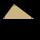# Triangle + quadratic equation - math problems

#### Number of problems found: 86

• Right triangleLegs of the right triangle are in the ratio a:b = 2:8. The hypotenuse has a length of 87 cm. Calculate the perimeter and area of the triangle.
• Triangle ABCTriangle ABC has side lengths m-1, m-2, m-3. What has to be m to be triangle a) rectangular b) acute-angled?
• TriangleCalculate the triangle sides if its area S = 630 and the second cathetus is shorter by 17.
• Right-angled triangleDetermine the content of a right triangle whose side lengths form successive members of an arithmetic progression and the radius of the circle described by the triangle is 5 cm.
• Rectangular triangleThe lengths of the rectangular triangle sides with a longer leg of 12 cm form an arithmetic sequence. What is the area of the triangle?
• Isosceles triangleThe leg of the isosceles triangle is 5 dm, its height is 20 cm longer than the base. Calculate base length z.
• Isosceles triangleIn an isosceles triangle ABC with base AB; A [3,4]; B [1,6] and the vertex C lies on the line 5x - 6y - 16 = 0. Calculate the coordinates of vertex C.
• CathetiThe hypotenuse of a right triangle is 41 and the sum of legs is 49. Calculate the length of its legs.
• RightDetermine angles of the right triangle with the hypotenuse c and legs a, b, if: ?
• The sidesThe sides of a right triangle form an arithmetic sequence. The hypotenuse is 24 cm long. Determine the remaining sides of the triangle.
• Right triangle eq2Hypotenuse of a right triangle is 9 cm longer than one leg and 8 cm longer than the second leg. Determine the circumference and area of a triangle.
• Three parallelsThe vertices of an equilateral triangle lie on 3 different parallel lines. The middle line is 5 m and 3 m distant from the end lines. Calculate the height of this triangle.
• Right triangle AlefThe obvod of a right triangle is 84 cm, and the hypotenuse is 37 cm long. Determine the lengths of the legs.
• An equilateralAn equilateral triangle is inscribed in a square of side 1 unit long so that it has one common vertex with the square. What is the area of the inscribed triangle?
• RT and circlesSolve right triangle if the radius of inscribed circle is r=9 and radius of circumscribed circle is R=23.
• Triangle ABC v2Area of the triangle is 12 cm square. Angle ACB = 30º , AC = (x + 2) cm, BC = x cm. Calculate the value of x.
• R triangleCalculate the right triangle area whose longer leg is 6 dm shorter than the hypotenuse and 3 dm longer than the shorter leg.
• Sphere from tree pointsEquation of sphere with three point (a,0,0), (0, a,0), (0,0, a) and center lies on plane x+y+z=a
• Difference of legsIn a right triangle, the length of the hypotenuse is 65 m, and the difference of legs is 23 m. Calculate the perimeter of this triangle.
• Right triangle eq2Find the lengths of the sides and the angles in the right triangle. Given area S = 210 and perimeter o = 70.

Do you have an interesting mathematical word problem that you can't solve it? Submit a math problem, and we can try to solve it.

We will send a solution to your e-mail address. Solved examples are also published here. Please enter the e-mail correctly and check whether you don't have a full mailbox.

Please do not submit problems from current active competitions such as Mathematical Olympiad, correspondence seminars etc...